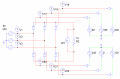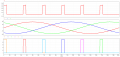# Doubt in the Concept of Full Wave Controlled Rectifier

Thread Starter

#### G Kartik Raja 1

Joined Nov 21, 2018
1
Consider a 3-phase fully controlled rectifier in which the thyristors are having a non-zero holding current (say 50mA). We know that the thyristors are triggered in a sequence with a phase delay of 60 deg, and at a time only one thyristor is triggered either from the postive group or from the negative group. So my question is how do any of the thyristors latch into conduction as we are turning ON only one thyristor either from the postive group or from the negative group?

In the lectures and books, they always consider ideal thyristors with zero holding current, so that the thyristor which has been triggered can remain in ON state until the other thyristor is triggered, which means, in such case, any two thyristors will remain ON all the time and we shall get a proper output voltage. But with non-zero holding current, it doesn't happen so.

This problem doesn't occur in single phase full wave rectifiers even with the non zero latching current being considered because we turn ON two thyristors at a time, one from the postive group and other from the negative group. So the thyristors can easily latch into conduction.

Simulation Graph when Latching Current is Considered (R Load, alpha = 30 deg)
The thyristors remain ON only during the time when their respective gate voltage is high, so we get a pulsed output voltage.#### ci139

Joined Jul 11, 2016
1,696
i guess the most energy transfer function for this rectifier could be different from simple Sin(t+0°)+Sin(t+120°)+Sin(t-120°)=0
i'm not into math.-ing it out right now -- but likely there should be ? 3 thyristors conducting at each point of time . . .

. . . a DC point check $$E_n=I·\left({r_n+R}\right)$$ since there are multiple sources $$\frac{\sum\ E_n-U}{\sum\ r_n}=I$$ (the least) has a 3 point solution where the R is best to add to r of the single source from one side . . . U @ junction R & two sources $$U_{3Pt}=\frac{E_1·r_2·r_3+E_2·\left({r_1+R}\right)·r_3+E_3·r_2·\left({r_1+R}\right)}{r_2·r_3+\left({r_1+R}\right)·r_3+r_2·\left({r_1+R}\right)}$$ → from where → $$U=I_1·R=\left({E_1-U_{3Pt}}\right)·\frac R{r_1+R}=\frac{E_1-U_{3Pt}}{\frac{r_1}R+1}$$ ← ← the point of this is to hint/remind that for parallel sources the composite r reduces

Last edited:

#### MrAl

Joined Jun 17, 2014
8,259
Consider a 3-phase fully controlled rectifier in which the thyristors are having a non-zero holding current (say 50mA). We know that the thyristors are triggered in a sequence with a phase delay of 60 deg, and at a time only one thyristor is triggered either from the postive group or from the negative group. So my question is how do any of the thyristors latch into conduction as we are turning ON only one thyristor either from the postive group or from the negative group?

In the lectures and books, they always consider ideal thyristors with zero holding current, so that the thyristor which has been triggered can remain in ON state until the other thyristor is triggered, which means, in such case, any two thyristors will remain ON all the time and we shall get a proper output voltage. But with non-zero holding current, it doesn't happen so.

This problem doesn't occur in single phase full wave rectifiers even with the non zero latching current being considered because we turn ON two thyristors at a time, one from the postive group and other from the negative group. So the thyristors can easily latch into conduction.

Simulation Graph when Latching Current is Considered (R Load, alpha = 30 deg)
The thyristors remain ON only during the time when their respective gate voltage is high, so we get a pulsed output voltage.
View attachment 191549

View attachment 191548
Hello,

You can not just turn one SCR on you have to turn at least two on or one on and hold the gate current until you turn the other one on.

#### DickCappels

Joined Aug 21, 2008
7,448
Good answer! For large SCRs you can drive the gate with a string of high frequency pulses. This is easier to do than driving the gates with DC in the case of the firing circuit being (transformer) isolated from the line.

Similar threads